# Perimeter of a rectangle

Go back to  'Perimeter'

 1 What is the Perimeter of a Rectangle? 2 Formula of Perimeter of a Rectangle 3 Important Notes on Rectangle 4 Solved Examples on Perimeter of a Rectangle 5 Tips and Tricks 6 Practice Questions on Perimeter of a Rectangle 7 Maths Olympiad Sample Papers 8 Frequently Asked Questions (FAQs)

We at Cuemath believe that Math is a life skill. Our Math Experts focus on the “Why” behind the “What.” Students can explore from a huge range of interactive worksheets, visuals, simulations, practice tests, and more to understand a concept in depth.

Book a FREE trial class today! and experience Cuemath’s LIVE Online Class with your child.

## What is the Perimeter of a Rectangle?

### Definition of the Perimeter of a Rectangle

The perimeter of a rectangle is the total length or distance of its boundary or all sides.

First let us understand the two main properties of a rectangle :

1. All four angles of a rectangle are 90 degrees.
2. Opposite sides of a rectangle are equal in length.

Imagine a rectangular park in your neighbourhood.

Have you ever thought about how long its boundary is?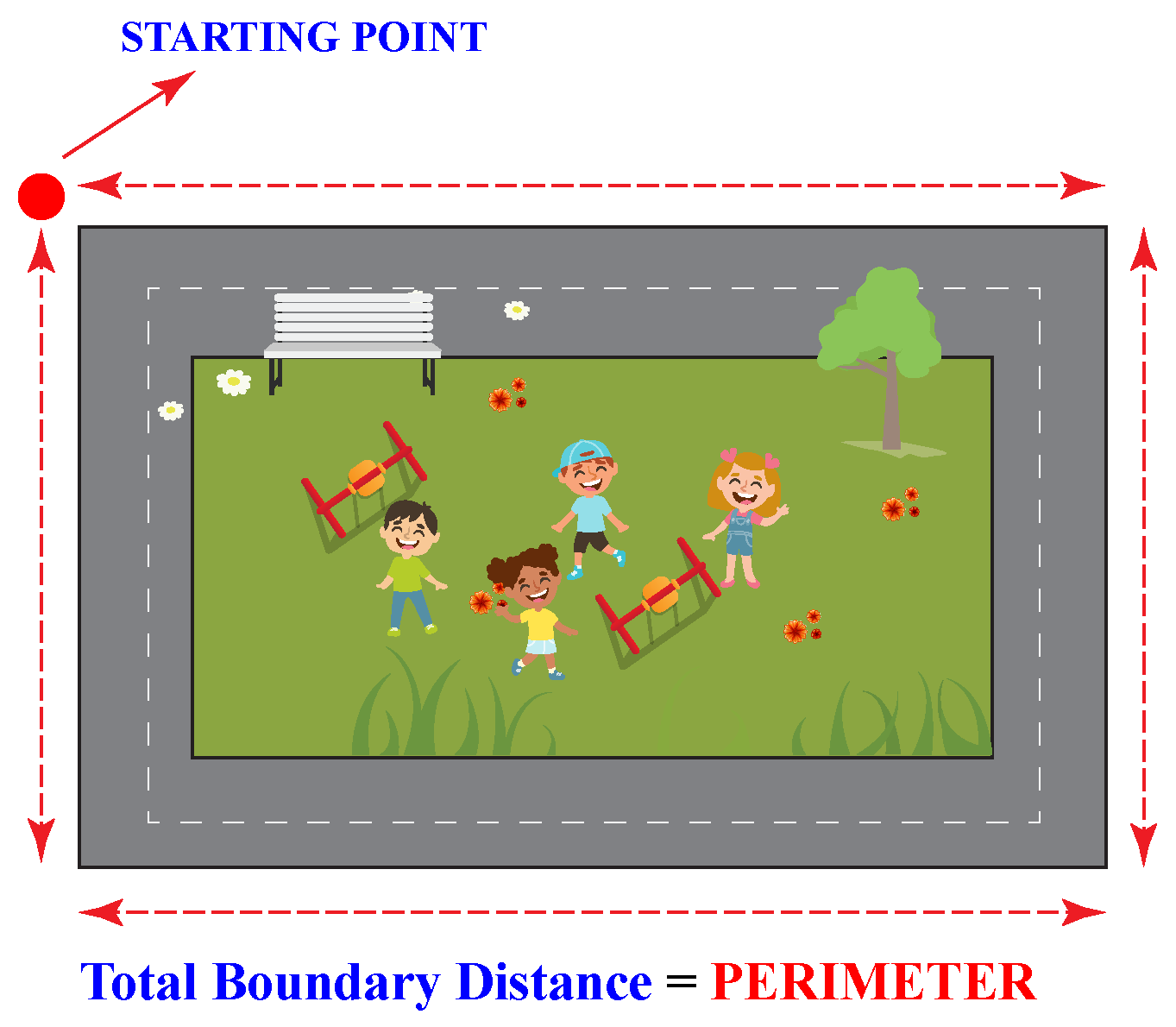If you go around the park along its boundary once, you cover a distance.

This distance is the perimeter of the park.

In order to measure the perimeter of any rectangular field, you travel along the boundary of the four sides of the field.

You begin at a fixed point called the starting point and end your round when you reach the starting point again.

This length or distance of the boundary is called the Perimeter of a rectangle.

## Formula of Perimeter of a Rectangle

The formula used to calculate the perimeter of a rectangle is:

 \begin{align}\text{Perimeter of a rectangle} = 2(l+b) \end{align}

Let us now understand this formula with an example.

David wants to put a fence around his farm so that his sheep will not wander away.

He wants to know how much wire he would need to put a fence around his rectangular farm.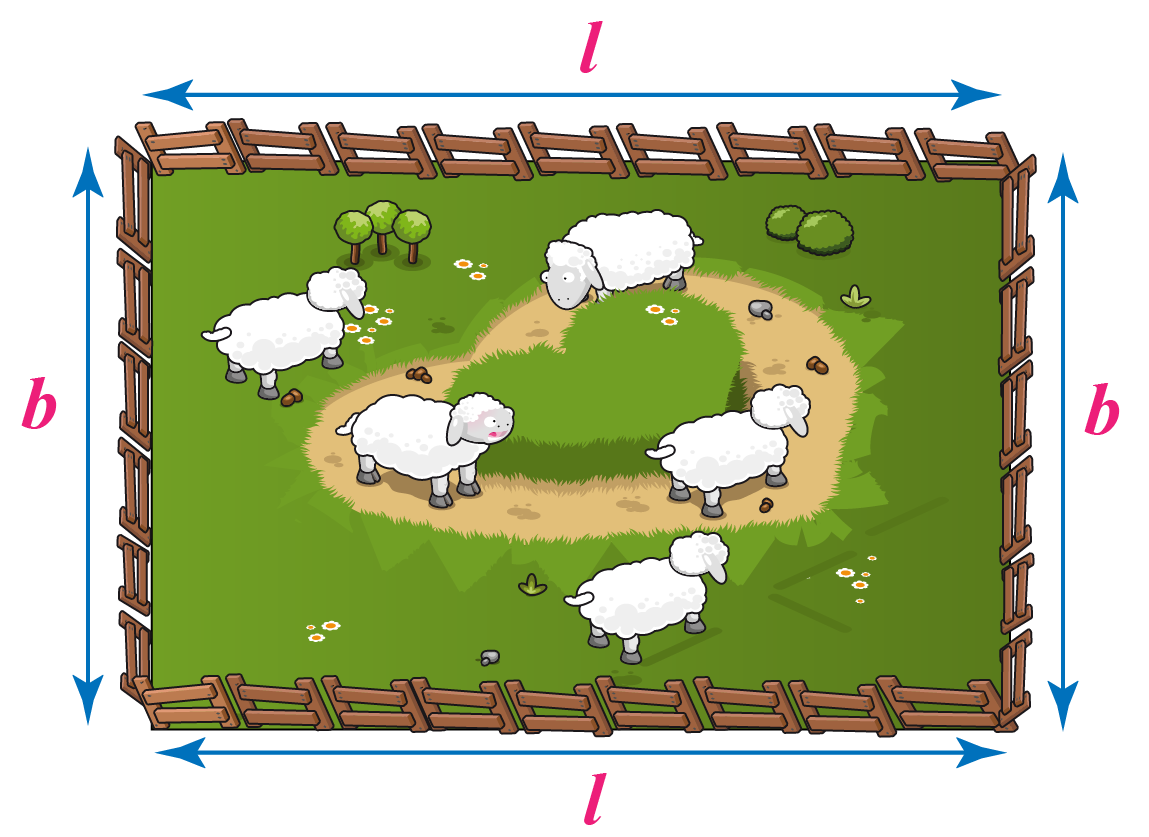Let us first name the sides of his farm.

The larger side of this rectangular farm is named $$l$$.

The smaller side is named $$b$$.

Now, if we add the distance of all the 4 sides of his farm, it will give us the perimeter.

Total distance $$= l + b + l + b = 2l + 2b$$

Therefore,

\begin{align}\text{Perimeter of a rectangle} = 2(l+b) \end{align}

• If the perimeter and the length of a rectangle are known, the breadth can be calculated using the formula:
 \begin{align}\text{Breadth}(b) &= \frac{\text{P}}{2} -l \end{align}
• In the same way, if the perimeter and the breadth are known, the length can be calculated using the formula:
 \begin{align}\text{Length} (l) &= \frac{\text{P}}{2} -b\end{align}

Use the following simulation to observe the change in the perimeter when the sides of a rectangle vary.

You can move the slider to change the length and breadth of the figure.

Help your child score higher with Cuemath’s proprietary FREE Diagnostic Test. Get access to detailed reports, customised learning plans and a FREE counselling session. Attempt the test now.

## Solved Examples :

 Example 1

Ria wants to add some beautiful lace as decoration to the borders of her doll's bedsheet.

The bedsheet is in the shape of a rectangle.

The length of the bedsheet is 120 cm and breadth is 85 cm.

How much lace will she need to put around it?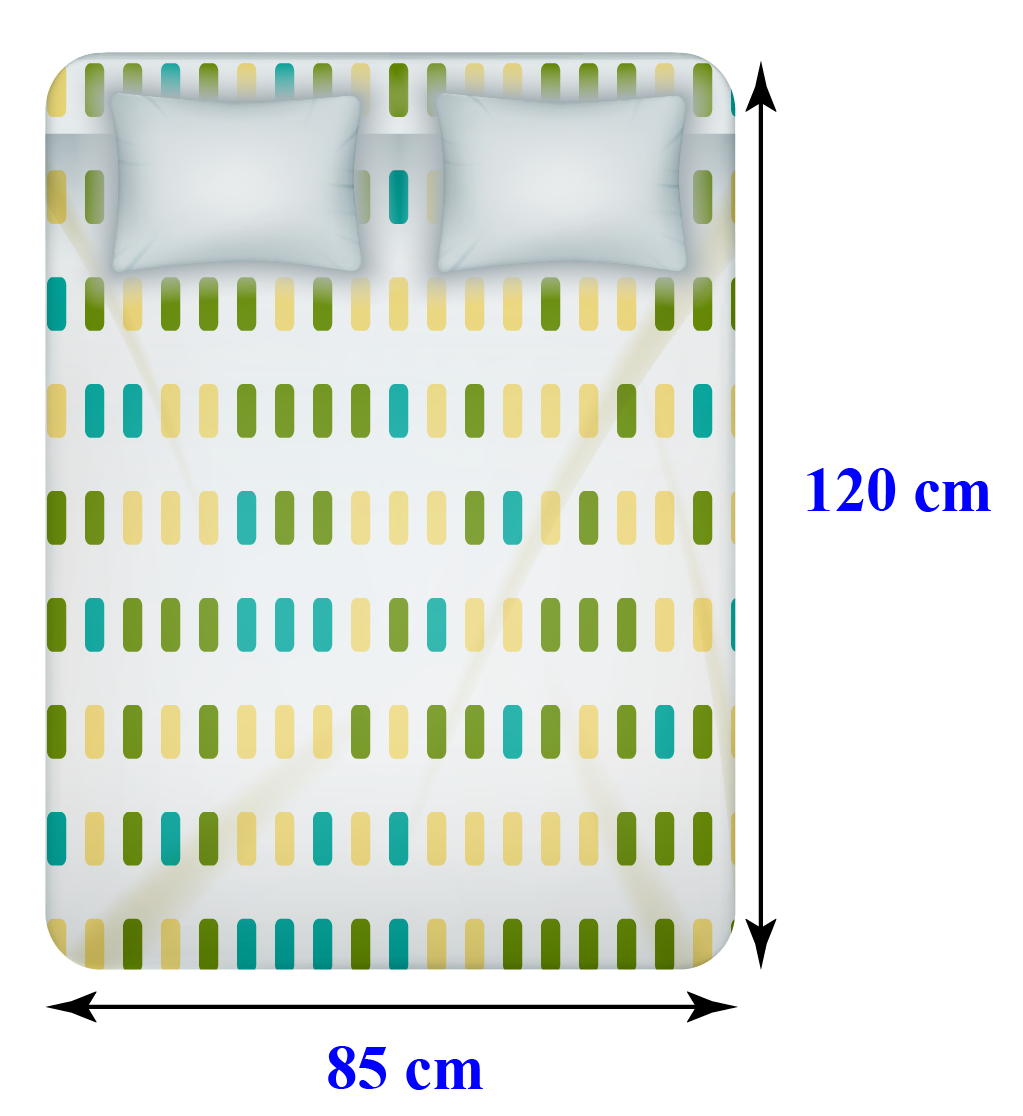Solution:

We know that:

\begin{align}\text{Perimeter of a rectangle} = 2(l+b) \end{align}

Given length $$l$$ = 120 cm; breadth $$b$$ = 85 cm

\begin{align} \text{Perimeter} &= 2(l+b) \\ &= 2 (120 + 85)\\ &= 2 \times205 \\ &= 410 \:\text{cm} \end{align}

 $$\therefore$$ Ria will need 410 cm of lace.
 Example 2

Your favourite chocolate bar is made up of equal-sized squares with each side measuring 1 cm.

Calculate its perimeter.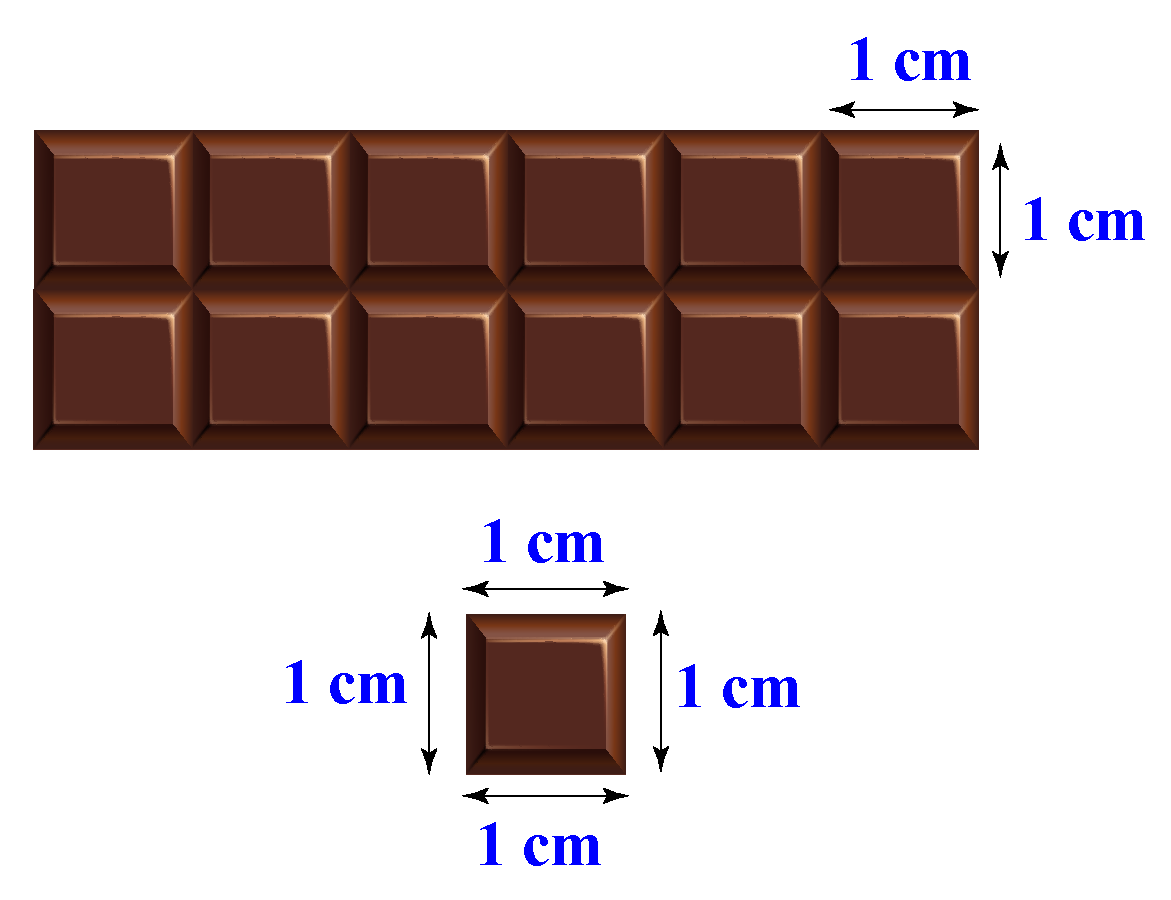Solution: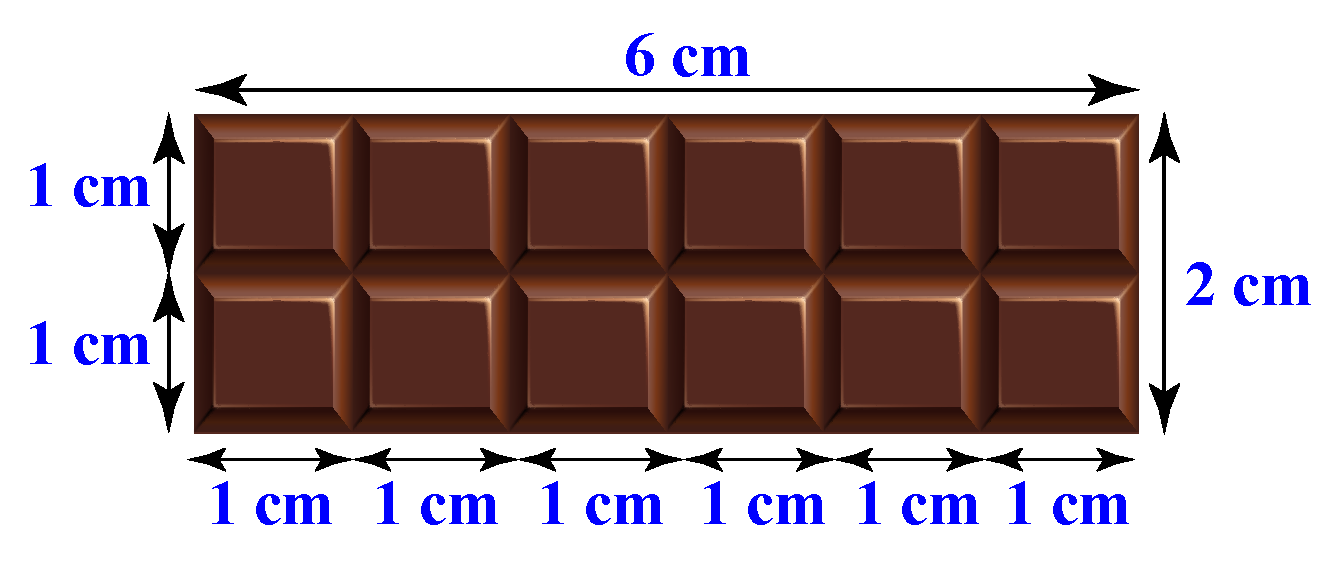We know that each little square has all its sides = 1 cm

If we count and add the sides of squares along the length of the bar, we get 6 cm.

The sides of squares along the breadth of the bar add upto 2 cm.

Therefore, the length of the bar = 6 cm

Breadth of the bar = 2 cm

\begin{align} \text{Perimeter} &= 2(l+b) \\ &= 2 (6+2)\\ &= 2 \times8 \\ &= 16 \:\text{cm} \end{align}

 $$\therefore$$ Perimeter of the chocolate bar is 16 cm.
 Example 3

Sam's coach makes him run around the basketball court twice to warm up before their basketball practice.

The court is 28 metres long and 15 metres wide.

What is the total distance that Sam runs everyday before practice?

Solution: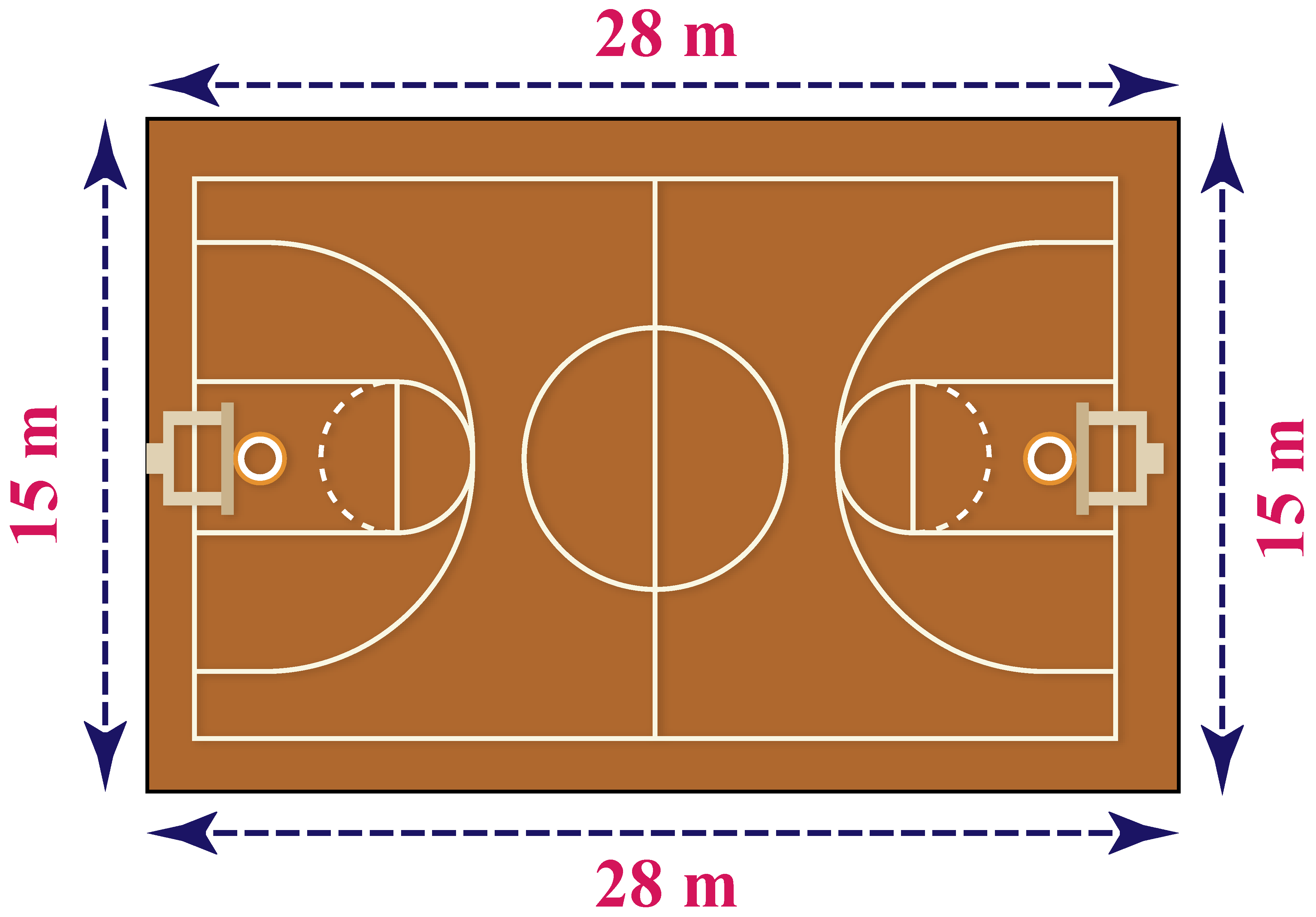Since Sam runs around all the sides of this basketball court, we need to find the perimeter of the court.

Length $$l$$ of the basketball court = 28 metres

Breadth $$b$$ of the basketball court = 15 metres

\begin{align} \text{Perimeter of a rectangle} &= 2(l+b)\\ &= 2 (28+15)\\ &= 2 \times43 \\ &= 86 \:\text{metres} \end{align}

We know that Sam runs around the court twice.

Hence, we multiply the perimeter by 2 :

$2 \times 86 =172 \:\text{m}$

 $$\therefore$$ Sam runs 172 metres.
 Example 4

The length of a door is 220 cm.

The breadth is 100 cm shorter than its length.

What is the perimeter of the door?

Solution: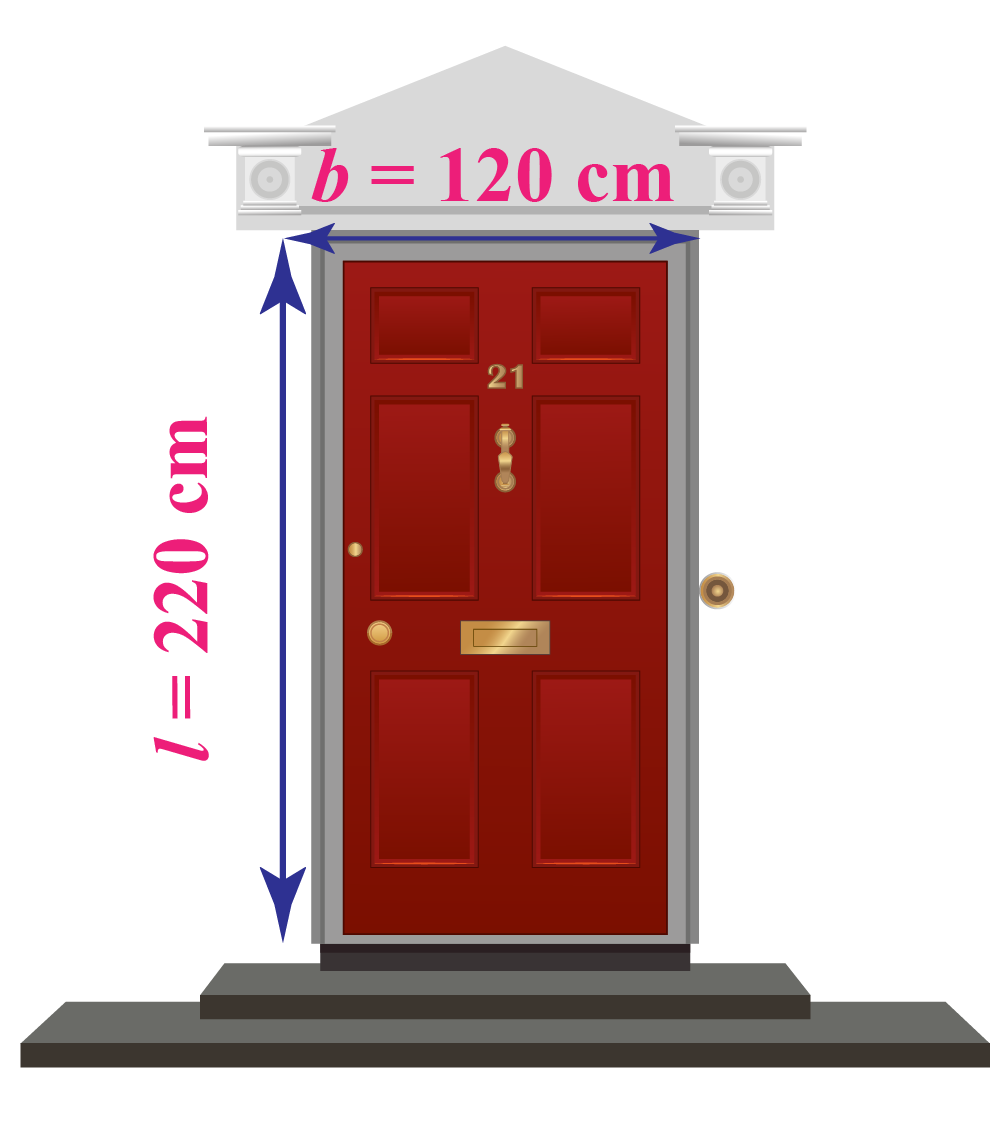Length of the door = 220 cm

We know that the breadth is 100 cm shorter than its length.

Breadth = 220-100 cm = 120 cm

\begin{align} \text{Perimeter} &= 2(l+b) \\ &= 2 (220+120)\\ &= 2 \times340 \\ &= 680 \:\text{cm} \end{align}

 $$\therefore$$ the perimeter of the door is 680 cm
 Example 5

The perimeter (P) of a rectangular garden is 48 metres and breadth (b) is 10 metres.

Find the length (l) of the garden.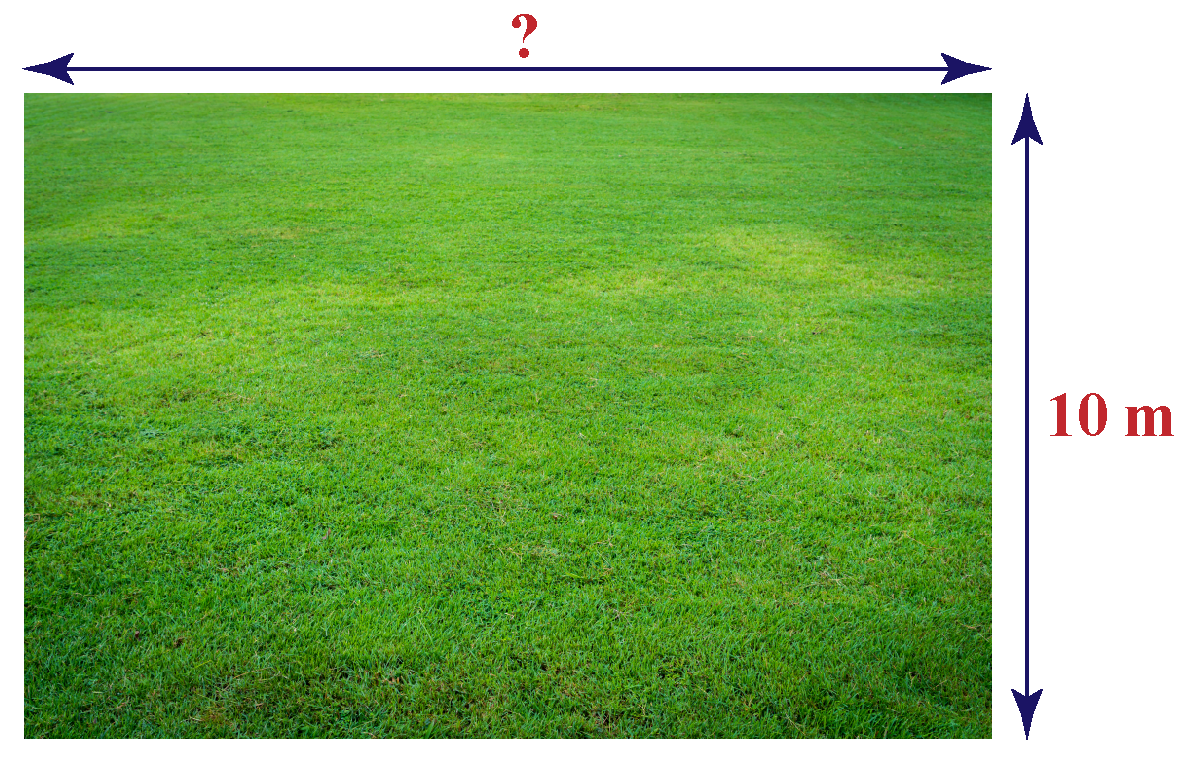Solution:

\begin{align} \text{Length}(l) &= \frac{\text{(P)}}{2} -b \\ &= \frac{48}{2} - 10 \\ &= 24 -10 \\ &= 14\: \text{m} \end{align}

 $$\therefore$$ the length of the garden is 14 mTips and Tricks
1. Do not forget to mention the unit along with the number. If the length of a rectangle is 7 cm, do not write just 7
2. While calculating the perimeter of a rectangle, make sure that all the dimensions (length and breadth) are in the same unit. For example, if the length is given in centimetres and breadth is given in metres, you need to change all the units to either metres or centimetres, as required.

Have a doubt that you want to clear? Get it clarified with simple solutions on Perimeter of a rectangle from our Math Experts at Cuemath’s LIVE, Personalised and Interactive Online Classes.

Make your kid a Math Expert, Book a FREE trial class today!

## Practice Questions

Try the Questions for Perimeter of a rectangle in the worksheet given below.

IMO (International Maths Olympiad) is a competitive exam in Mathematics conducted annually for school students. It encourages children to develop their math solving skills from a competition perspective.

## 1. What is the area and perimeter of a rectangle?

The area of a rectangle is the number of unit squares that can fit into it.

The definition of the perimeter of a rectangle is the total length or distance of its boundary or all sides.

In other words, perimeter refers to the sum of all its sides.

## 2. How to find the perimeter of a rectangle?

The perimeter of a rectangle can be calculated if the length $$l$$ and breadth $$b$$ of the rectangle are known.

The values are placed in the formula : \begin{align}\text{Perimeter of a rectangle} = 2(l+b) \end{align}

## 3. What is the formula for the perimeter of a rectangle?

The formula for calculating the perimeter of a rectangle is : \begin{align}\text{Perimeter of a rectangle} = 2(l+b) \end{align}, where $$l$$ is the length of the rectangle and $$b$$ is the breadth of the rectangle.

More Important Topics
Numbers
Algebra
Geometry
Measurement
Money
Data
Trigonometry
Calculus